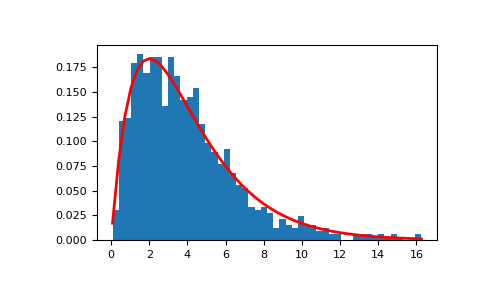# numpy.random.gamma¶

random.gamma(shape, scale=1.0, size=None)

Draw samples from a Gamma distribution.

Samples are drawn from a Gamma distribution with specified parameters, shape (sometimes designated “k”) and scale (sometimes designated “theta”), where both parameters are > 0.

Note

New code should use the gamma method of a default_rng() instance instead; please see the Quick Start.

Parameters
shapefloat or array_like of floats

The shape of the gamma distribution. Must be non-negative.

scalefloat or array_like of floats, optional

The scale of the gamma distribution. Must be non-negative. Default is equal to 1.

sizeint or tuple of ints, optional

Output shape. If the given shape is, e.g., (m, n, k), then m * n * k samples are drawn. If size is None (default), a single value is returned if shape and scale are both scalars. Otherwise, np.broadcast(shape, scale).size samples are drawn.

Returns
outndarray or scalar

Drawn samples from the parameterized gamma distribution.

scipy.stats.gamma

probability density function, distribution or cumulative density function, etc.

Generator.gamma

which should be used for new code.

Notes

The probability density for the Gamma distribution is

$p(x) = x^{k-1}\frac{e^{-x/\theta}}{\theta^k\Gamma(k)},$

where $$k$$ is the shape and $$\theta$$ the scale, and $$\Gamma$$ is the Gamma function.

The Gamma distribution is often used to model the times to failure of electronic components, and arises naturally in processes for which the waiting times between Poisson distributed events are relevant.

References

1

Weisstein, Eric W. “Gamma Distribution.” From MathWorld–A Wolfram Web Resource. http://mathworld.wolfram.com/GammaDistribution.html

2

Wikipedia, “Gamma distribution”, https://en.wikipedia.org/wiki/Gamma_distribution

Examples

Draw samples from the distribution:

>>> shape, scale = 2., 2.  # mean=4, std=2*sqrt(2)
>>> s = np.random.gamma(shape, scale, 1000)


Display the histogram of the samples, along with the probability density function:

>>> import matplotlib.pyplot as plt
>>> import scipy.special as sps
>>> count, bins, ignored = plt.hist(s, 50, density=True)
>>> y = bins**(shape-1)*(np.exp(-bins/scale) /
...                      (sps.gamma(shape)*scale**shape))
>>> plt.plot(bins, y, linewidth=2, color='r')
>>> plt.show()